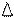Chapter 10.3, Problem 30EElementary Geometry For College St...

7th Edition
Alexander + 2 others
ISBN: 9781337614085

Solutions

Chapter
SectionElementary Geometry For College St...

7th Edition
Alexander + 2 others
ISBN: 9781337614085
Textbook ProblemThe drawing shows parallelogram R S T V .a) What type of number is r ?b) Find an expression for R S .c) Describe the coordinate t in terms of the other variables shown.To determine

(a)

To find:

The type of number r using the given parallelogram.

Explanation

The given figure is shown below.

To determine

(b)

To find:

The expression for RS.

To determine

(c)

To describe:

The variable t in terms of other variables.

Still sussing out bartleby?

Check out a sample textbook solution.

See a sample solution

The Solution to Your Study Problems

Bartleby provides explanations to thousands of textbook problems written by our experts, many with advanced degrees!

Get Started

Show by means of an example that limxa[f(x)g(x)] may exist even though neither limxaf(x) nor limxag(x)

Applied Calculus for the Managerial, Life, and Social Sciences: A Brief Approach

In Problems 33-38, use inverse matrices to find the solution of the systems of equations. 37.

Mathematical Applications for the Management, Life, and Social Sciences

Solve the equations in Exercises 112 for x (mentally, if possible). x+5=0

Finite Mathematics and Applied Calculus (MindTap Course List)

Evaluate the integral. 01coshtdt

Single Variable Calculus: Early Transcendentals